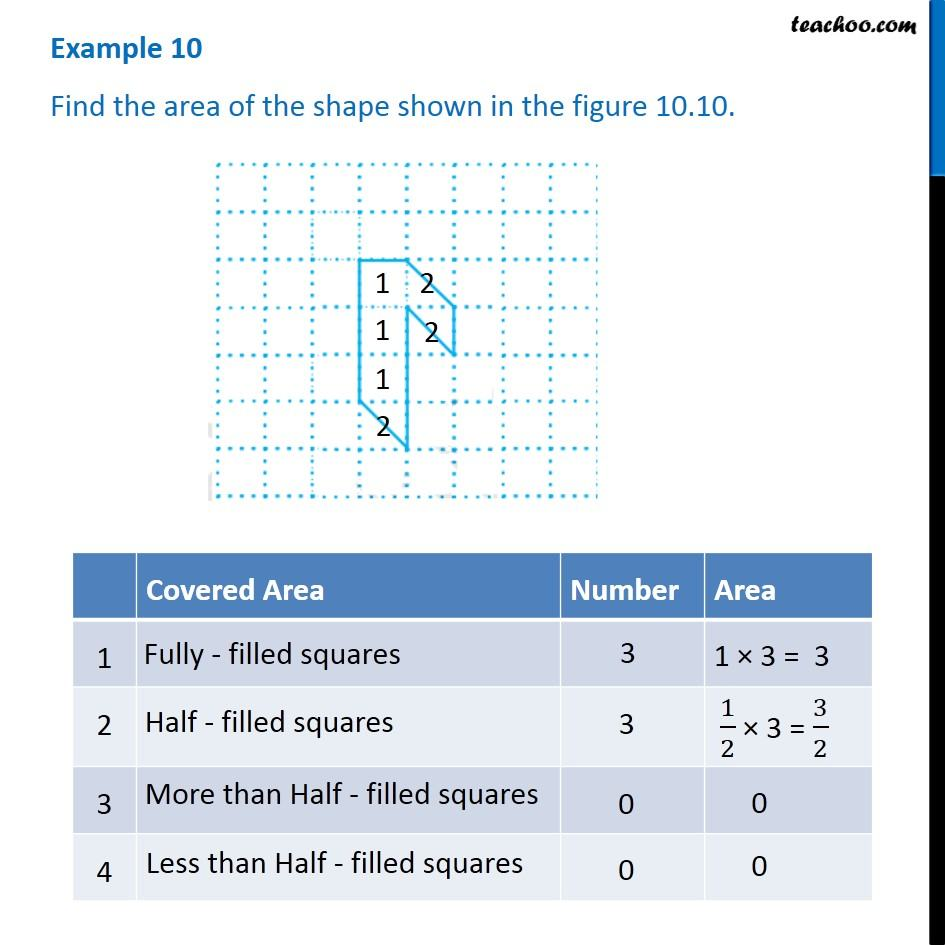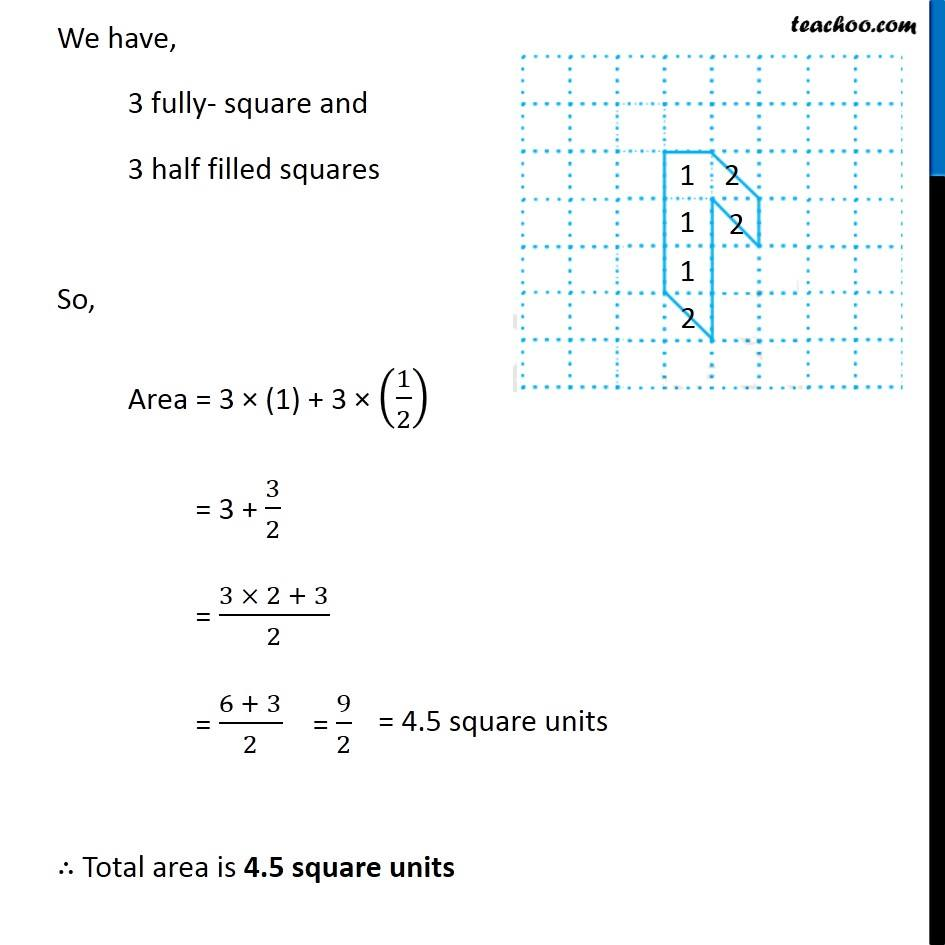1. Chapter 10 Class 6 Mensuration
2. Concept wise
3. Finding area by counting squares

Transcript

Example 10 Find the area of the shape shown in the figure 10.10. We have, 3 fully- square and 3 half filled squares So, Area = 3 × (1) + 3 × (1/2) = 3 + 3/2 = (3 × 2 + 3)/2 = (6 + 3)/2 = 9/2 = 4.5 square units ∴ Total area is 4.5 square units